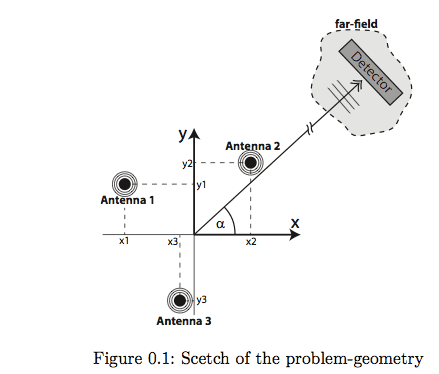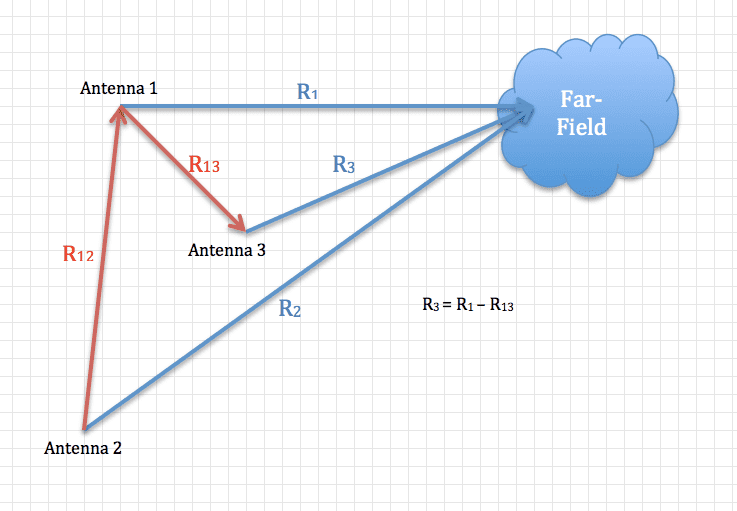# Far-field power-pattern of the antenna array Beam-Steering

• banigeo
In summary, the antenna array maximizes the power at a selected angle when the phase of the individual antennas are manipulated to create a pattern. The maximum power is at α = 0 degrees.f

#### banigeo

Hi all,
I have a problem to firstly understand and secondly solve this problem :
here is the Problem :
Consider three (coherent) antennas variously arranged on the x-y-plane where each individual antenna radiates an isotropic electric field E(x).
1 - Write down an expression for the far-field power-pattern of the antenna array in the x-y-plane.
2 - Configure the phase of each individual antenna in such a way, that the resulting power-pattern of the antenna-array will have a maximum at a chosen angle α .
3 - plot the normalised power-pattern as polar-plot both in natural- and logarithmic scale for at least two different look-angles.## Homework Equations

Ei(r,t) = E0(xi)ei(k.r - ωt + kxi sin(θ))

## The Attempt at a Solution

[/B]
Z = 0

#### Attachments

Last edited:
Hi all,
I have a problem to firstly understand and secondly solve this problem :
here is the Problem :
Consider three (coherent) antennas variously arranged on the x-y-plane where each individual antenna radiates an isotropic electric field E(x).
1 - Write down an expression for the far-field power-pattern of the antenna array in the x-y-plane.
2 - Configure the phase of each individual antenna in such a way, that the resulting power-pattern of the antenna-array will have a maximum at a chosen angle α .
3 - plot the normalised power-pattern as polar-plot both in natural- and logarithmic scale for at least two different look-angles.

View attachment 77547

## Homework Equations

Ei(r,t) = E0(xi)ei(k.r - ωt + kxi sin(θ))

## The Attempt at a Solution

[/B]
Z = 0

Welcome to the PF.

Can you please show an attempt at a solution? We require that before we can be of much tutorial help.

You should certainly be able to write the phase differences for the sources to maximize the power at the RX antenna.

Welcome to the PF.

Can you please show an attempt at a solution? We require that before we can be of much tutorial help.

You should certainly be able to write the phase differences for the sources to maximize the power at the RX antenna.
Hi Berkeman,

here is some solution, which I tried and also there is some more tips about question :Epw=E0 ei(k.x - wt)
E0=1 , pw=Plane Wave
all of them have the maximum amplitude = 1

E1=ei(k.x - wt)=1
E3=ei(k.r3-wt)=ei(kr1-wt)e-ikr13

what is k ? >>> k=k.e^α

Thank You,